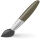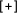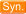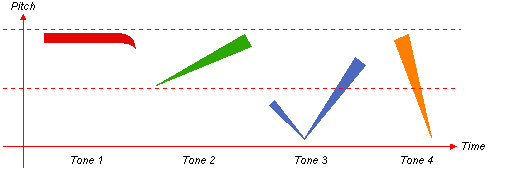"(math.)"  Chinese-English DictionaryDICTIONARY OPTIONS
 配方 pèi fāng prescription / cooking recipe / formulation / completing the square (to solve quadratic equation, math)高数 gāo shù further math / advanced mathematics (school subject, abbr. for 高等數學|高等数学)有理数 yǒu lǐ shù rational number (i.e. fraction of two integers, math)一一映射 yī yī yìng shè bijective map (i.e. map between sets in math. that is one-to-one and onto) / one-to-one correspondence公因式 gōng yīn shì common factor / common divisor (of a math. expression)因式 yīn shì factor / divisor (of a math. expression)奇解 qí jiě singular solution (to a math. equation)特解 tè jiě particular solution (to a math. equation)配方法 pèi fāng fǎ completing the square (method of solving quadratic equation, math)